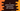# How can we access global variables in C### How can we access global variables in C:

Global variables are variables in C those are accessible from any method. Local variables are accessible only within the function where defined. For example, let’s consider the below example program:

``````#include <stdio.h>

void anotherFunction()
{
int result = 30;
printf("result in anotherFunction = %d\n", result);
}
int main()
{
int result = 20;
printf("result in main = %d\n", result);
anotherFunction();
}``````

Here, we have the main function and another function called anotherFunction. We are calling anotherFunction from main. Notice that we have two variables with same name result are defined in these functions.

If you run this program, it will first print the value of result in main and then it will print the result in anotherFunction. Both of these variables result are local variables. So, their scopes are limited inside their own function where they are defined.

But the scope of a global variable is not limited to a function. Global variables are defined outside of all functions and it can be accessed from all other functions. But, how can we access a global variable from a function ? For example, if we create one variable result as global variable, how can we access it from main or anotherFunction ?

It is actually easy. We need to use one keyword called extern for that. Let me show you how.

### extern keyword to access global variables in C:

Using extern keyword, we can access a global variable in C. We need to redeclare the variable using extern in the function where we are accessing it. extern actually tells the compiler that it is a global variable and it is already defined.

Let’s take a look at the below example program:

``````#include <stdio.h>
int result = 100;

int main()
{
int result = 20;

{
extern int result;
printf("Global result = %d\n",result);
}

printf("result in main = %d\n", result);
}``````

It will print the below output:

``````Global result = 100
result in main = 20``````

So, you can see that we are printing the value of both result variables. One is local and another is global.

Also, you don’t need an extra block if your global variable’s name is different than local :

``````#include <stdio.h>
int globalVar = 100;

int main()
{
int result = 20;
extern int globalVar;
printf("Global result = %d\n", globalVar);

printf("result in main = %d\n", result);
}``````